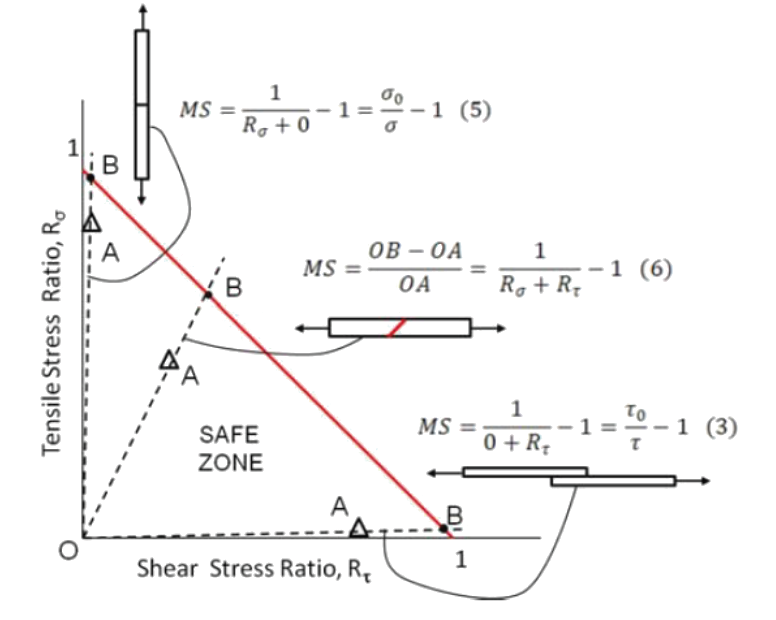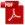### 12.4.1. Practical Joint Strength and Accounting for Variability

Reference: Abbott, Richard. Analysis and Design of Composite and Metallic Flight Vehicle Structures 3 Edition, 2019

This level of joint strength depends on the braze being ‘perfect’. The work that Flom has done at NASA covers the interaction of direct and shear load effects and gives a simple assumption to cover the likely quality variability of the brazed joint.

Where:

The test results used for this figure are determined by test in the following way.Figure 12.4.1‑2: Graphical Representation of Margin of Safety for Butt Brazed, Scarf and Lap Shear Brazed Joints  (NASA 20120008328, 2012)

Determination of appropriate allowable shear strength values is given as follows:  (NASA 20120008193, 2012) gives the following guidance for the relationship between brazed strength test mean values and B-basis strength:

The effective B-basis brazed strength should be assumed to be 0.5 of the test mean strength for both tension strength and shear strength.

If it can be assumed that the pristine brazed joint develops the same strength of the parent material, preliminary margins of safety at ultimate load level can be generated with the following expression:

Where Ftu  and Fsu  are the material strength of the parent material. Note that the normalized strength of the parent material should be used.

Note: This analysis method is approximate only. All critical joint strengths must be based on relevant test data.

Note:  (MIL-HNDBK-5H, 1998) section 8.2 and  (NASA TM X-73305, 1975) section B 1.3.1 and B 1.3.2 states that the allowable shear strength used for both copper and silver brazed joints in steel alloys should be limited to 15ksi. If the brazing is done without particular process control the following modified and conservative margin of safety for shear strength should be used:

Complete list of brazing references at the Abbott Aerospace Technical Library:

### 12.4.1. Practical Joint Strength and Accounting for Variability

Reference: Abbott, Richard. Analysis and Design of Composite and Metallic Flight Vehicle Structures 3 Edition, 2019

This level of joint strength depends on the braze being ‘perfect’. The work that Flom has done at NASA covers the interaction of direct and shear load effects and gives a simple assumption to cover the likely quality variability of the brazed joint.

Where:

The test results used for this figure are determined by test in the following way.Figure 12.4.1‑2: Graphical Representation of Margin of Safety for Butt Brazed, Scarf and Lap Shear Brazed Joints  (NASA 20120008328, 2012)

Determination of appropriate allowable shear strength values is given as follows:  (NASA 20120008193, 2012) gives the following guidance for the relationship between brazed strength test mean values and B-basis strength:

The effective B-basis brazed strength should be assumed to be 0.5 of the test mean strength for both tension strength and shear strength.

If it can be assumed that the pristine brazed joint develops the same strength of the parent material, preliminary margins of safety at ultimate load level can be generated with the following expression:

Where Ftu  and Fsu  are the material strength of the parent material. Note that the normalized strength of the parent material should be used.

Note: This analysis method is approximate only. All critical joint strengths must be based on relevant test data.

Note:  (MIL-HNDBK-5H, 1998) section 8.2 and  (NASA TM X-73305, 1975) section B 1.3.1 and B 1.3.2 states that the allowable shear strength used for both copper and silver brazed joints in steel alloys should be limited to 15ksi. If the brazing is done without particular process control the following modified and conservative margin of safety for shear strength should be used: